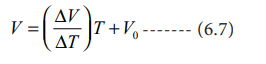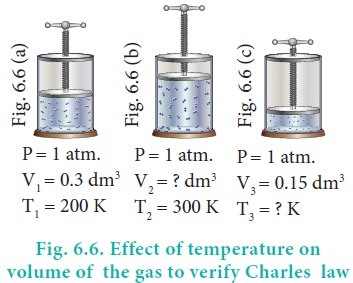Home | | Chemistry 11th std | Charles Law (Volume-temperature relationship)

# Charles Law (Volume-temperature relationship)

The relationship between volume of a gas and its temperature was examined by J. A. C. Charles.

Charles Law (Volume-temperature relationship)

The relationship between volume of a gas and its temperature was examined by J. A. C. Charles. He observed that for a fixed mass of a gas at constant pressure, the volume is directly proportional to its temperature (K). Mathematically it can be represented as (at constant P and n)

or V = kT ------- (6.5)

or V/T = Constant

If the temperature of the gas increases, the volume also increases in direct proportion, so that V/T is a constant.

For the same system at constant pressure, one can writeFor example, if a balloon is moved from an ice cold water bath to a boiling water bath, the temperature of the gas increases. As a result, the gas molecules inside the balloon move faster and gas expands. Hence, the volume increases.### Variation of volume with temperature at constant pressure

The plot of the volume of the gas against its temperature at a given pressure  is shown in the figure 6.5. From the graph it is clear that the volume of the gas linearly increases with temperature at a given pressure. Such lines are called isobars. It can be expressed by the following straight line equation.

V = mT + C where T is the temperature in degree Celsius and m & C are constants.

When T= 0 ┬║C the volume becomes V0. Hence, V0 = C and slope of the straight line m is equal to ╬öV / ╬öT. Therefore the above equation can be written in the following form.(n, P are constant)

Divide the equation 6.7 by VoCharles and Gay Lussac found that under constant pressure, the relative increase in volume per degree increase in temperature is same for all gases. The relative increase in volume per ┬║ C (╬▒) is equal to 1/V0(ŌłåV/ŌłåT)

ThereforeCharles found that the coefficient of expansion is approximately equal to 1/273. It means that at constant temperature for a given mass, for each degree rise in temperature, all gases expand by 1/273 of their volume at 0 0CIf we extrapolate the straight line in the figure 6.5 beyond the experimental measurements, the straight line intersects the temperature axis (x-axis) at -2730 C. This shows that the volume of the gas becomes zero at -2730 C, more precisely this temperature is -273.150 C. Beyond this temperature the gas would have a negative volume which is physically impossible. For this reason, this temperature was defined as absolute zero by Kelvin and he proposed a new temperature scale with absolute zero as starting point which is now called Kelvin scale. The only difference between the Kelvin scale of temperature and Celsius scale of temperature is that the zero position is shifted. The boiling and freezing point of water in both scales are given below.### Example:

In figure 6.6 let us find the missing parameters (volume in 6.6 (b) and temperature in 6.6(c))Tags : The Gas Laws , 11th Chemistry : UNIT 6 : Gaseous State
Study Material, Lecturing Notes, Assignment, Reference, Wiki description explanation, brief detail
11th Chemistry : UNIT 6 : Gaseous State : Charles Law (Volume-temperature relationship) | The Gas Laws